Download Presentation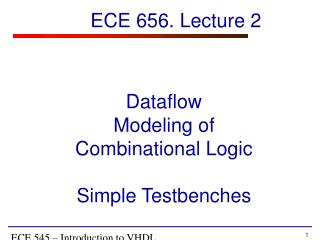Data f low Modeling of Combinational Logic Simple Testbenches

# Data f low Modeling of Combinational Logic Simple Testbenches - PowerPoint PPT Presentation

Data f low Modeling of Combinational Logic Simple Testbenches. ECE 656. Lecture 2. Resources. Volnei A. Pedroni , Circuit Design with VHDL Chapter 5, Concurrent Code (without 5.5) Chapter 4.1, Operators Sundar Rajan, Essential VHDL: RTL Synthesis Done RightI am the owner, or an agent authorized to act on behalf of the owner, of the copyrighted work described.
Download Presentation## Data f low Modeling of Combinational Logic Simple Testbenches

An Image/Link below is provided (as is) to download presentation

Download Policy: Content on the Website is provided to you AS IS for your information and personal use and may not be sold / licensed / shared on other websites without getting consent from its author.While downloading, if for some reason you are not able to download a presentation, the publisher may have deleted the file from their server.

- - - - - - - - - - - - - - - - - - - - - - - - - - E N D - - - - - - - - - - - - - - - - - - - - - - - - - -
Presentation Transcript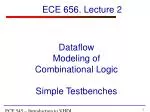Dataflow Modeling of Combinational LogicSimple Testbenches

ECE 656. Lecture 2

ECE 545 – Introduction to VHDL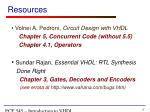ECE 545 – Introduction to VHDLResources
• Volnei A. Pedroni,Circuit Design with VHDL

Chapter 5, Concurrent Code (without 5.5)

Chapter 4.1, Operators

• Sundar Rajan, Essential VHDL: RTL Synthesis

Done Right

Chapter 3, Gates, Decoders and Encoders

(see errata at http://www.vahana.com/bugs.htm)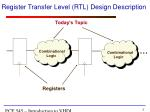ECE 545 – Introduction to VHDLRegister Transfer Level (RTL) Design Description

Combinational

Logic

Combinational

Logic

Today’s Topic

Registers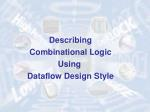ECE 545 – Introduction to VHDL

Describing

Combinational Logic

Using

Dataflow Design Style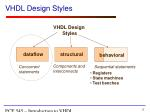ECE 545 – Introduction to VHDLVHDL Design Styles

dataflow

VHDL Design

Styles

structural

behavioral

Components and

interconnects

Concurrent

statements

Sequential statements

• Registers
• State machines
• Test benches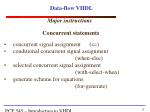ECE 545 – Introduction to VHDL

Data-flow VHDL

Major instructions

Concurrent statements

• concurrent signal assignment ()
• conditional concurrent signal assignment

(when-else)

• selected concurrent signal assignment

(with-select-when)

• generate scheme for equations

(for-generate)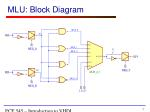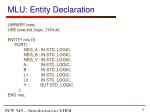ECE 545 – Introduction to VHDLMLU: Entity Declaration

LIBRARY ieee;

USE ieee.std_logic_1164.all;

ENTITY mlu IS

PORT(

NEG_A : IN STD_LOGIC;

NEG_B : IN STD_LOGIC;

NEG_Y : IN STD_LOGIC;

A : IN STD_LOGIC;

B : IN STD_LOGIC;

L1 : IN STD_LOGIC;

L0 : IN STD_LOGIC;

Y : OUT STD_LOGIC

);

END mlu;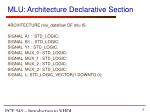ECE 545 – Introduction to VHDLMLU: Architecture Declarative Section

ARCHITECTURE mlu_dataflow OF mlu IS

SIGNAL A1 : STD_LOGIC;

SIGNAL B1 : STD_LOGIC;

SIGNAL Y1 : STD_LOGIC;

SIGNAL MUX_0 : STD_LOGIC;

SIGNAL MUX_1 : STD_LOGIC;

SIGNAL MUX_2 : STD_LOGIC;

SIGNAL MUX_3 : STD_LOGIC;

SIGNAL L:STD_LOGIC_VECTOR(1 DOWNTO 0);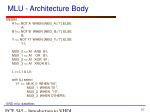ECE 545 – Introduction to VHDLMLU - Architecture Body

BEGIN

A1<=NOT A WHEN (NEG_A='1') ELSE

A;

B1<=NOT B WHEN (NEG_B='1') ELSE

B;

Y<=NOT Y1 WHEN (NEG_Y='1') ELSE

Y1;

MUX_0<=A1 AND B1;

MUX_1<=A1 OR B1;

MUX_2<=A1 XOR B1;

MUX_3<=A1 XNOR B1;

L <= L1 & L0;

with (L) select

Y1<=MUX_0 WHEN "00",

MUX_1 WHEN "01",

MUX_2 WHEN "10",

MUX_3 WHEN OTHERS;

END mlu_dataflow;ECE 545 – Introduction to VHDL

Data-flow VHDL

Major instructions

Concurrent statements

• concurrent signal assignment ()
• conditional concurrent signal assignment

(when-else)

• selected concurrent signal assignment

(with-select-when)

• generate scheme for equations

(for-generate)ECE 545 – Introduction to VHDLFor Generate Statement

For - Generate

label:FORidentifier IN rangeGENERATE

BEGIN

{Concurrent Statements}

END GENERATE;ECE 545 – Introduction to VHDLPARITY: Entity Declaration

LIBRARYieee;

USEieee.std_logic_1164.all;

ENTITYparityIS

PORT(

parity_in : IN STD_LOGIC_VECTOR(7 DOWNTO 0);

parity_out : OUT STD_LOGIC

);

ENDparity;ECE 545 – Introduction to VHDLPARITY: Block Diagram

xor_out(1)

xor_out(2)

xor_out(3)

xor_out(4)

xor_out(5)

xor_out(6)ECE 545 – Introduction to VHDLPARITY: Architecture

ARCHITECTUREparity_dataflowOFparityIS

SIGNALxor_out: std_logic_vector (6 downto 1);

BEGIN

xor_out(1) <= parity_in(0) XORparity_in(1);

xor_out(2) <= xor_out(1) XORparity_in(2);

xor_out(3) <= xor_out(2) XORparity_in(3);

xor_out(4) <= xor_out(3) XORparity_in(4);

xor_out(5) <= xor_out(4) XORparity_in(5);

xor_out(6) <= xor_out(5) XORparity_in(6);

parity_out <= xor_out(6) XORparity_in(7);

ENDparity_dataflow;ECE 545 – Introduction to VHDLPARITY: Block Diagram (2)

xor_out(0)

xor_out(1)

xor_out(2)

xor_out(3)

xor_out(4)

xor_out(5)

xor_out(6)

xor_out(7)ECE 545 – Introduction to VHDLPARITY: Architecture

ARCHITECTUREparity_dataflowOFparityIS

SIGNALxor_out: STD_LOGIC_VECTOR (7 downto 0);

BEGIN

xor_out(0) <= parity_in(0);

xor_out(1) <= xor_out(0) XORparity_in(1);

xor_out(2) <= xor_out(1) XORparity_in(2);

xor_out(3) <= xor_out(2) XORparity_in(3);

xor_out(4) <= xor_out(3) XORparity_in(4);

xor_out(5) <= xor_out(4) XORparity_in(5);

xor_out(6) <= xor_out(5) XORparity_in(6);

xor_out(7) <= xor_out(6) XORparity_in(7);

parity_out <= xor_out(7);

ENDparity_dataflow;ECE 545 – Introduction to VHDLPARITY: Architecture (2)

ARCHITECTUREparity_dataflowOFparityIS

SIGNALxor_out: STD_LOGIC_VECTOR (7 DOWNTO 0);

BEGIN

xor_out(0) <= parity_in(0);

G2: FOR i IN1TO7GENERATE

xor_out(i) <= xor_out(i-1) XORparity_in(i);

end generate G2;

parity_out <= xor_out(7);

ENDparity_dataflow;ECE 545 – Introduction to VHDL

Combinational Logic Synthesis

for

BeginnersECE 545 – Introduction to VHDL

Simple rules for beginners

For combinational logic,

use only concurrent statements

• concurrent signal assignment ()
• conditional concurrent signal assignment

(when-else)

• selected concurrent signal assignment

(with-select-when)

• generate scheme for equations

(for-generate)ECE 545 – Introduction to VHDL

Simple rules for beginners

For circuits composed of

- simple logic operations (logic gates)

- simple arithmetic operations (addition,

subtraction, multiplication)

- shifts/rotations by a constant

use

• concurrent signal assignment ()ECE 545 – Introduction to VHDL

Simple rules for beginners

For circuits composed of

- multiplexers

- decoders, encoders

- tri-state buffers

use

• conditional concurrent signal assignment

(when-else)

• selected concurrent signal assignment

(with-select-when)ECE 545 – Introduction to VHDL

Left vs. right side of the assignment

Left side

<=

<= when-else

with-select <=

Right side

Expressions including:

• Internal signals (defined

in a given architecture)

• Ports of the mode

- in

- inout

- buffer

• Internal signals (defined

in a given architecture)

• Ports of the mode

- out

- inout

- bufferECE 545 – Introduction to VHDLArithmetic operations

Synthesizable arithmetic operations:

Subtraction, -

Comparisons, >, >=, <, <=

Multiplication, *

Division by a power of 2, /2**6(equivalent to right shift)

Shifts by a constant, SHL, SHRECE 545 – Introduction to VHDLArithmetic operations

The result of synthesis of an arithmetic

operation is a

- combinational circuit

- without pipelining.

The exact internal architecture used

(and thus delay and area of the circuit)

may depend on the timing constraints specified

during synthesis (e.g., the requested maximum

clock frequency).ECE 545 – Introduction to VHDLOperations on Unsigned Numbers

For operations on unsigned numbers

USE ieee.std_logic_unsigned.all

and

signals (inputs/outputs) of the type

STD_LOGIC_VECTOR

OR

USE ieee.std_logic_arith.all

and

signals (inputs/outputs) of the type

UNSIGNEDECE 545 – Introduction to VHDLOperations on Signed Numbers

For operations on signed numbers

USE ieee.std_logic_signed.all

and

signals (inputs/outputs) of the type

STD_LOGIC_VECTOR

OR

USE ieee.std_logic_arith.all

and

signals (inputs/outputs) of the type

SIGNEDECE 545 – Introduction to VHDLSigned and Unsigned Types

Behave exactly like

STD_LOGIC_VECTOR

plus, they determine whether a given vector

should be treated as a signed or unsigned number.

Require

USE ieee.std_logic_arith.all;ECE 545 – Introduction to VHDLAddition of Unsigned Numbers

LIBRARY ieee ;

USE ieee.std_logic_1164.all ;

USE ieee.std_logic_unsigned.all ;

PORT ( Cin : IN STD_LOGIC ;

X, Y : IN STD_LOGIC_VECTOR(15 DOWNTO 0) ;

S : OUT STD_LOGIC_VECTOR(15 DOWNTO 0) ;

Cout : OUT STD_LOGIC ) ;

ARCHITECTURE Behavior OF adder16 IS

SIGNAL Sum : STD_LOGIC_VECTOR(16 DOWNTO 0) ;

BEGIN

Sum <= ('0' & X) + Y + Cin ;

S <= Sum(15 DOWNTO 0) ;

Cout <= Sum(16) ;

END Behavior ;ECE 545 – Introduction to VHDLAddition of Unsigned Numbers

LIBRARY ieee ;

USE ieee.std_logic_1164.all ;

USE ieee.std_logic_arith.all ;

PORT ( Cin : IN STD_LOGIC ;

X, Y : IN UNSIGNED(15 DOWNTO 0) ;

S : OUT UNSIGNED(15 DOWNTO 0) ;

Cout : OUT STD_LOGIC ) ;

ARCHITECTURE Behavior OF adder16 IS

SIGNAL Sum : UNSIGNED(16 DOWNTO 0) ;

BEGIN

Sum <= ('0' & X) + Y + Cin ;

S <= Sum(15 DOWNTO 0) ;

Cout <= Sum(16) ;

END Behavior ;ECE 545 – Introduction to VHDLAddition of Signed Numbers (1)

LIBRARY ieee ;

USE ieee.std_logic_1164.all ;

USE ieee.std_logic_signed.all ;

PORT ( Cin : IN STD_LOGIC ;

X, Y : IN STD_LOGIC_VECTOR(15 DOWNTO 0) ;

S : OUT STD_LOGIC_VECTOR(15 DOWNTO 0) ;

Cout, Overflow : OUT STD_LOGIC ) ;

ARCHITECTURE Behavior OF adder16 IS

SIGNAL Sum : STD_LOGIC_VECTOR(16 DOWNTO 0) ;

BEGIN

Sum <= ('0' & X) + Y + Cin ;

S <= Sum(15 DOWNTO 0) ;

Cout <= Sum(16) ;

Overflow <= Sum(16) XOR X(15) XOR Y(15) XOR Sum(15) ;

END Behavior ;ECE 545 – Introduction to VHDLAddition of Signed Numbers (2)

LIBRARY ieee ;

USE ieee.std_logic_1164.all ;

USE ieee.std_logic_arith.all ;

PORT ( Cin : IN STD_LOGIC ;

X, Y : IN SIGNED(15 DOWNTO 0) ;

S : OUT SIGNED(15 DOWNTO 0) ;

Cout, Overflow : OUT STD_LOGIC ) ;

ARCHITECTURE Behavior OF adder16 IS

SIGNAL Sum : SIGNED(16 DOWNTO 0) ;

BEGIN

Sum <= ('0' & X) + Y + Cin ;

S <= Sum(15 DOWNTO 0) ;

Cout <= Sum(16) ;

Overflow <= Sum(16) XOR X(15) XOR Y(15) XOR Sum(15) ;

END Behavior ;ECE 545 – Introduction to VHDLMultiplication of signed and unsigned numbers (1)

LIBRARY ieee;

USE ieee.std_logic_1164.all;

USE ieee.std_logic_arith.all ;

entity multiply is

port(

a : in STD_LOGIC_VECTOR(15 downto 0);

b : in STD_LOGIC_VECTOR(7 downto 0);

cu : out STD_LOGIC_VECTOR(23 downto 0);

cs : out STD_LOGIC_VECTOR(23 downto 0)

);

end multiply;

architecture dataflow of multiply is

SIGNAL sa: SIGNED(15 downto 0);

SIGNAL sb: SIGNED(7 downto 0);

SIGNAL sres: SIGNED(23 downto 0);

SIGNAL ua: UNSIGNED(15 downto 0);

SIGNAL ub: UNSIGNED(7 downto 0);

SIGNAL ures: UNSIGNED(23 downto 0);ECE 545 – Introduction to VHDLMultiplication of signed and unsigned numbers (2)

begin

-- signed multiplication

sa <= SIGNED(a);

sb <= SIGNED(b);

sres <= sa * sb;

cs <= STD_LOGIC_VECTOR(sres);

-- unsigned multiplication

ua <= UNSIGNED(a);

ub <= UNSIGNED(b);

ures <= ua * ub;

cu <= STD_LOGIC_VECTOR(ures);

end dataflow;ECE 545 – Introduction to VHDLInteger Types

Operations on signals (variables)

of the integer types:

INTEGER, NATURAL,

and their sybtypes, such as

TYPE day_of_month IS RANGE 0 TO 31;

are synthesizable in the range

-(231-1) .. 231 -1 for INTEGERs and their subtypes

0 .. 231 -1 for NATURALs and their subtypesECE 545 – Introduction to VHDLInteger Types

Operations on signals (variables)

of the integer types:

INTEGER, NATURAL,

are less flexible and more difficult to control

than operations on signals (variables) of the type

STD_LOGIC_VECTOR

UNSIGNED

SIGNED, and thus

are recommened to be avoided by beginners.ECE 545 – Introduction to VHDLAddition of Signed Integers

PORT ( X, Y : IN INTEGER RANGE -32767 TO 32767 ;

S : OUT INTEGER RANGE -32767 TO 32767 ) ;

ARCHITECTURE Behavior OF adder16 IS

BEGIN

S <= X + Y ;

END Behavior ;ECE 545 – Introduction to VHDLGenerating selected values of one input

SIGNAL test_vector : STD_LOGIC_VECTOR(2 downto 0);

BEGIN

.......

testing: PROCESS

BEGIN

test_vector <= "000";

WAIT FOR 10 ns;

test_vector <= "001";

WAIT FOR 10 ns;

test_vector <= "010";

WAIT FOR 10 ns;

test_vector <= "011";

WAIT FOR 10 ns;

test_vector <= "100";

WAIT FOR 10 ns;

END PROCESS;

........

END behavioral;ECE 545 – Introduction to VHDLGenerating all values of one input

SIGNAL test_vector : STD_LOGIC_VECTOR(3 downto 0):="0000";

BEGIN

.......

testing: PROCESS

BEGIN

WAIT FOR 10 ns;

test_vector <= test_vector + 1;

end process TESTING;

........

END behavioral;ECE 545 – Introduction to VHDL

Generating all possible values of two inputs

SIGNAL test_ab : STD_LOGIC_VECTOR(1 downto 0);

SIGNAL test_sel : STD_LOGIC_VECTOR(1 downto 0);

BEGIN

.......

double_loop: PROCESS

BEGIN

test_ab <="00";

test_sel <="00";

for I in 0 to 3 loop

for J in 0 to 3 loop

wait for 10 ns;

test_ab <= test_ab + 1;

end loop;

test_sel <= test_sel + 1;

end loop;

END PROCESS;

........

END behavioral;ECE 545 – Introduction to VHDLGenerating periodical signals, such as clocks

CONSTANT clk1_period : TIME := 20 ns;

CONSTANT clk2_period : TIME := 200 ns;

SIGNAL clk1 : STD_LOGIC;

SIGNAL clk2 : STD_LOGIC := ‘0’;

BEGIN

.......

clk1_generator: PROCESS

clk1 <= ‘0’;

WAIT FOR clk1_period/2;

clk1 <= ‘1’;

WAIT FOR clk1_period/2;

END PROCESS;

clk2 <= not clk2after clk2_period/2;

.......

END behavioral;ECE 545 – Introduction to VHDLGenerating one-time signals, such as resets

CONSTANT reset1_width : TIME := 100 ns;

CONSTANT reset2_width : TIME := 150 ns;

SIGNAL reset1 : STD_LOGIC;

SIGNAL reset2 : STD_LOGIC := ‘1’;

BEGIN

.......

reset1_generator: PROCESS

reset1 <= ‘1’;

WAIT FOR reset_width;

reset1 <= ‘0’;

WAIT;

END PROCESS;

reset2_generator: PROCESS

WAIT FOR reset_width;

reset2 <= ‘0’;

WAIT;

END PROCESS;

.......

END behavioral;ECE 545 – Introduction to VHDLTypical error

SIGNAL test_vector : STD_LOGIC_VECTOR(2 downto 0);

SIGNAL reset : STD_LOGIC;

BEGIN

.......

generator1: PROCESS

reset <= ‘1’;

WAIT FOR 100 ns

reset <= ‘0’;

test_vector <="000";

WAIT;

END PROCESS;

generator2: PROCESS

WAIT FOR 200 ns

test_vector <="001";

WAIT FOR 600 ns

test_vector <="011";

END PROCESS;

.......

END behavioral;ECE 545 – Introduction to VHDLWait for vs. Wait

0

0

1

2

3

1

2

3

Wait for: waveform will keep repeating itself forever

Wait: waveform will keep its state after the last wait instruction.ECE 545 – Introduction to VHDLAssert

Assert is a non-synthesizable statement

whose purpose is to write out messages

on the screen when problems are found

during simulation.

Depending on the severity of the problem,

The simulator is instructed to continue

simulation or halt.ECE 545 – Introduction to VHDLAssert - syntax

ASSERT condition

[REPORT "message"

[SEVERITY severity_level ];

The message is written when the condition

is FALSE.

Severity_level can be:

Note, Warning, Error (default), or Failure.ECE 545 – Introduction to VHDLAssert - Examples

assert initial_value <= max_value

report "initial value too large"

severity error;

assert packet_length /= 0

report "empty network packet received"

severity warning;

assert false

report "Initialization complete"

severity „note”;ECE 545 – Introduction to VHDLReport - syntax

REPORT "message"

[SEVERITY severity_level ];

The message is always written.

Severity_level can be:

Note (default), Warning, Error, or Failure.ECE 545 – Introduction to VHDLReport - Examples

report "Initialization complete";

report "Current time = "& time'image(now);

report "Incorrect branch" severity error;ECE 545 – Introduction to VHDLGenerating reports in the message window

reports: process(clk_trigger) begin

if (clk_trigger = '0' and clk_trigger'EVENT) then

case segments is

when seg_0 => report time'image(now) & ": 0 is displayed" ;

when seg_1 => report time'image(now) & ": 1 is displayed" ;

when seg_2 => report time'image(now) & ": 2 is displayed" ;

when seg_3 => report time'image(now) & ": 3 is displayed" ;

when seg_4 => report time'image(now) & ": 4 is displayed" ;

when seg_5=> report time'image(now) & ": 5 is displayed" ;

when seg_6 => report time'image(now) & ": 6 is displayed" ;

when seg_7 => report time'image(now) & ": 7 is displayed" ;

when seg_8 => report time'image(now) & ": 8 is displayed" ;

when seg_9 => report time'image(now) & ": 9 is displayed" ;

end case;

end if;

end process;ECE 545 – Introduction to VHDLAnatomy of a Process

[label:] process[(sensitivity list)]

[declaration part]

begin

statement part

end process;ECE 545 – Introduction to VHDLSequential Statements (1)

If statement

else and elsifare optional

ifboolean expression then

statements

elsif boolean expression then

statements

else boolean expression then

statements

end if;ECE 545 – Introduction to VHDLSequential Statements (3)

Loop Statement

Repeats a section of VHDL code

fori in range loop

statements

end loop;ECE 545 – Introduction to VHDLSequential Statements (2)

Case statement

Choices have to cover all possible values of the condition

Use others to specify all remaining cases

casecondition is

when choice_1 =>

statements

when choice_2 =>

statements

when others =>

statements

end case;